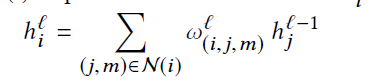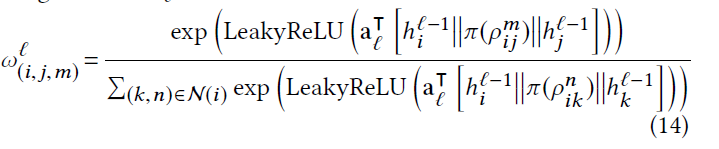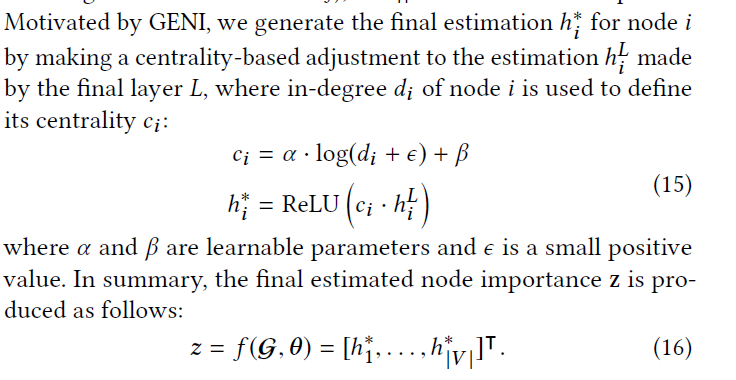## 简介：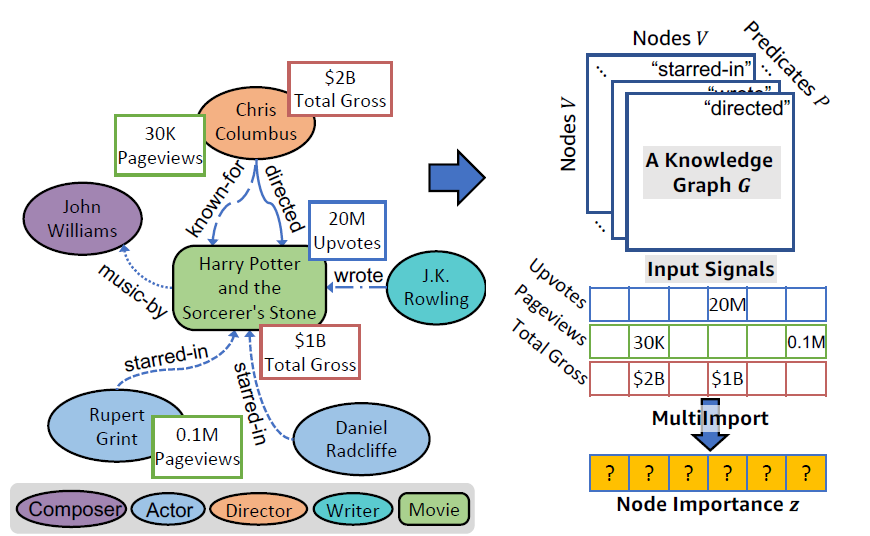1. 节点的重要性以不同的属性作为评价，所得到的结果是不一样的。
2. 可能会存在空属性的情况，导致节点在那个属性下无法评价重要性。

## 符号定义

### Node Feature

$X\in \mathbb{R}^{|V|\times F}$

### Node Importance

$z\in \mathbb{R}_{\geq 0}$，是一个非负的实数，代表图中每个节点的重要性。

### Input Signal

$V’ \to \mathbb{R}_{\geq 0}(V’ \subseteq V)$，同样也是一个重要性，但是一个部分的映射，把一些具有相同属性节点映射到一个重要性空间。对一个数据源，可能有多种不同的signals，比如一本书可以有销售的数量，销售的金额，投票的数量等，所以对一本书的受欢迎的评价可能来自多个不同的方面。同样一些signal只对一些种类的节点有效，所以是一个部分映射。

• 输入的信号有不同的尺度，可能一个值域是在0-1之间，另一个是在0-100之间。
• 输入的信号都是值越大节点越重要。

## Object Function

$p(\theta)$,是先验参数分布，作者用了中值为0的高斯分布，

$p(\mathcal{G}|\theta)$是用来给定参数$\theta$来表达图的概率。给定一个节点，将其用函数$g(\cdot)$嵌入到低纬空间，然后用KG的triplet来表达图。公式如下：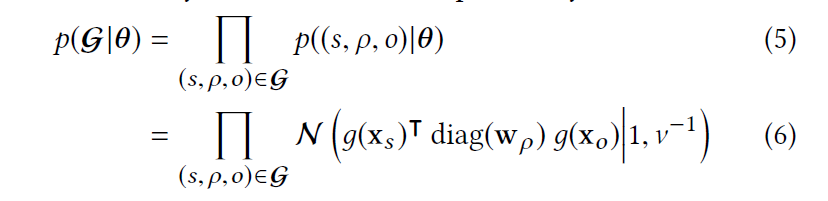$p(S|\mathcal{G},\theta)$是给定一个图和参数，每一个信号出现的概率，其假设学习出的参数，能拟合节点在所有signal里的重要性概率，用极大似然概率，得到如下公式：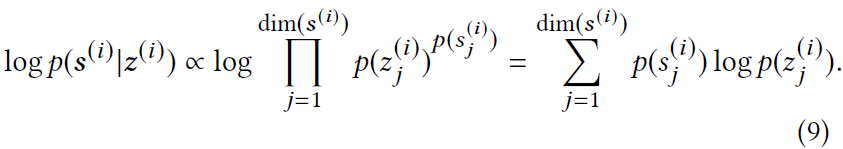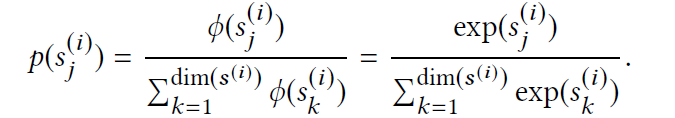$p(z_j^{(i)})$则是我们学习到的重要性，其表示如下：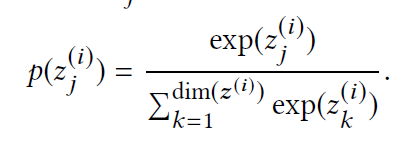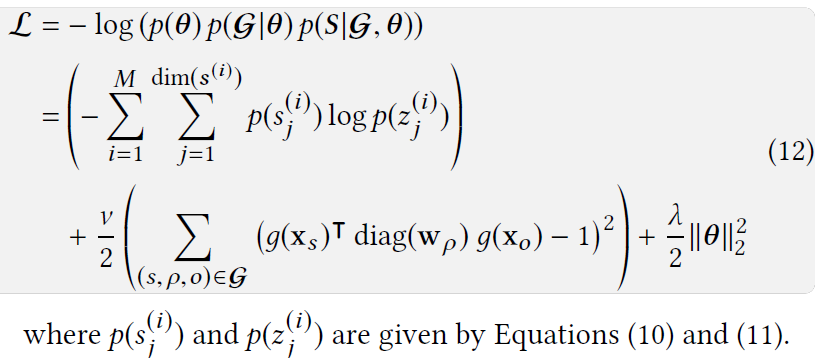## 模型和训练过程